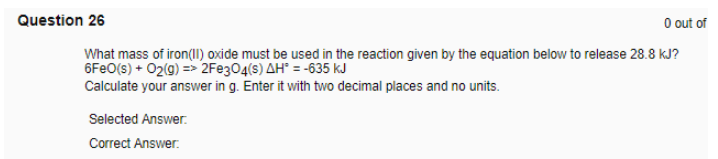# Problem: What mass of iron(II) oxide must be used in the reaction given by the equation below to release 28.8 kJ? 6FeO (s) + O2 (g) = &gt; 2Fe3O4 (s)    ΔH° = -635 kJ Calculate your answer in g. Enter it with two decimal places and no units.

###### FREE Expert Solution
98% (289 ratings)###### Problem Details

What mass of iron(II) oxide must be used in the reaction given by the equation below to release 28.8 kJ?

6FeO (s) + O2 (g) = > 2Fe3O4 (s)    ΔH° = -635 kJ

Calculate your answer in g. Enter it with two decimal places and no units.